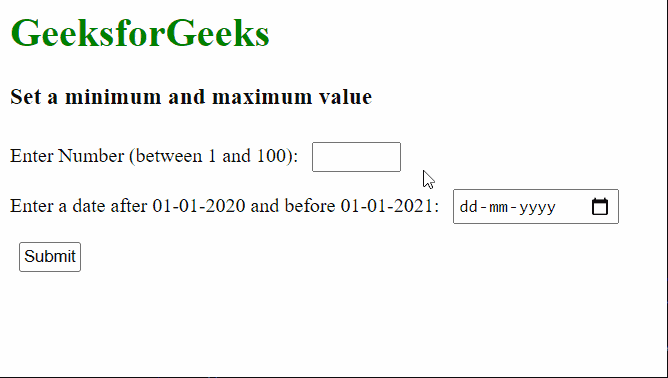# How to set a minimum and maximum value for an input element in HTML5 ?

• Last Updated : 17 Mar, 2021

In this article, we are going to learn how to set a minimum and maximum value for an input element by using the HTML <input> min and max attribute. This prevents the input element from accepting values that are lower than the minimum value or higher than the maximum.

Approach: This can be implemented by using the min and max attributes:

• min: This attribute accepts a minimum value for the input element.
• max: This attribute accepts a minimum value for the input element.

These parameters together can be used to specify a range of numbers that can be accepted as input. Note that these attributes only work on the input type of number and date.

Syntax:

`<input type="number" min="min_value" max="max_value">`

Example: In this example, we have used two inputs with different input types, one is a number type and the other is the date type. The minimum and maximum range of the number is from 1 to 100 and the dates are from 01-01-2020 to 01-01-2021.

## HTML

 `<``html``> ` `<``head``> ` `  ``<``style` `type``=``"text/css"``> ` `    ``label { ` `      ``font: 18px; ` `    ``} ` ` `  `    ``input { ` `      ``margin: 7px; ` `      ``padding: 2px ` `    ``} ` `  `` ` ` ` `<``body``> ` `  ``<``h1` `style``=``"color: green"``> ` `    ``GeeksforGeeks ` `  `` ` `  ``<``h3``>Set a minimum and maximum value ` `  ``<``form``> ` `    ``<``label` `for``=``"Number"``> ` `      ``Enter Number (between 1 and 100): ` `    `` ` `    ``<``input` `type``=``"number"` `id``=``"Number"`  `           ``name``=``"Number"` `min``=``"1"` `max``=``"100"``> ` `    ``<``br``> ` `    ``<``label` `for``=``"datemin"``>Enter a date after ` `      ``01-01-2020 and before 01-01-2021: ` `    `` ` `    ``<``input` `type``=``"date"` `id``=``"datemin"` `name``=``"datemin"` `           ``min``=``"2020-01-01"` `max``=``"2021-01-01"``> ` `    ``<``br``> ` `    ``<``input` `type``=``"submit"``> ` `  `` ` ` ` ``

Output:My Personal Notes arrow_drop_up
Recommended Articles
Page :